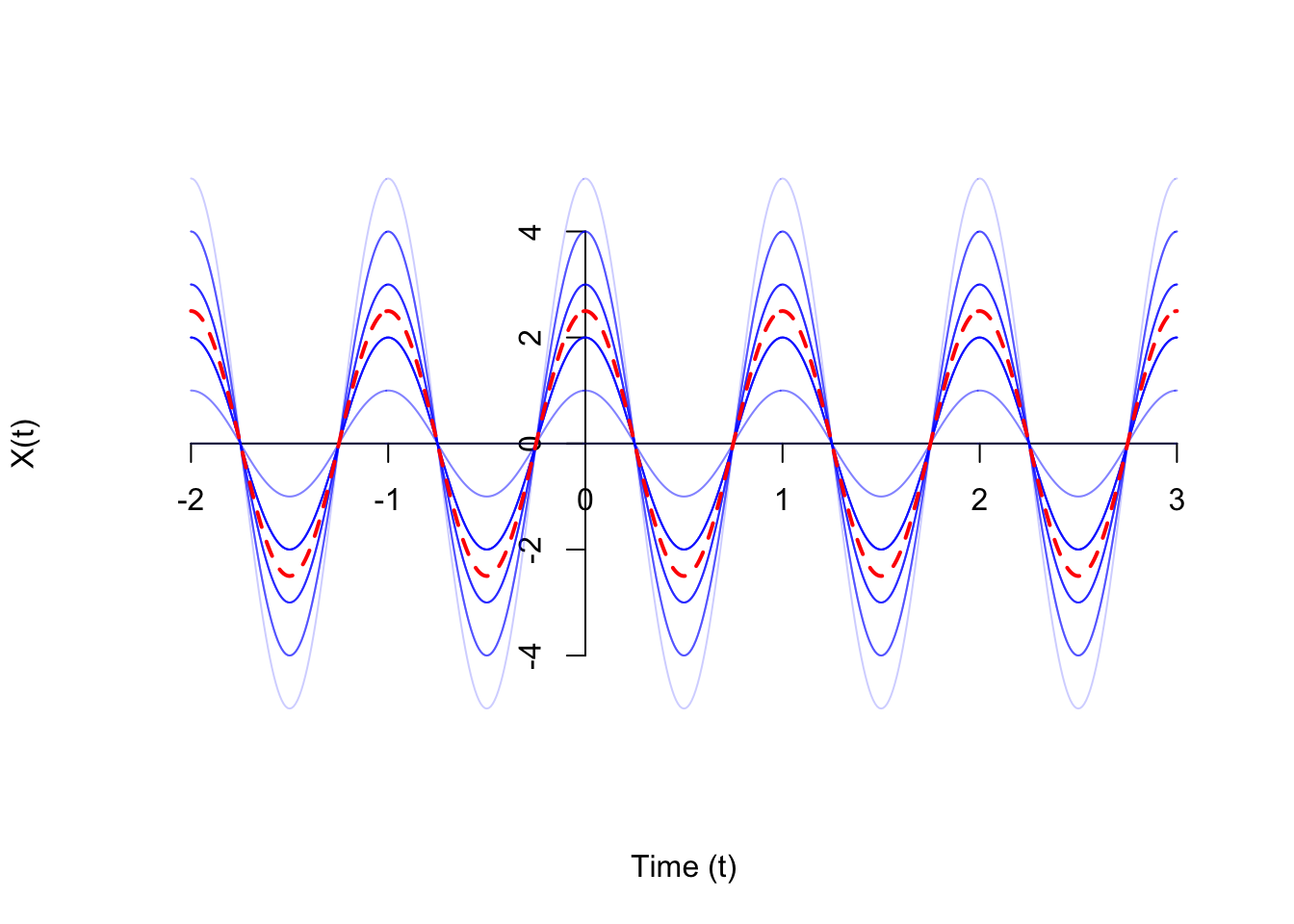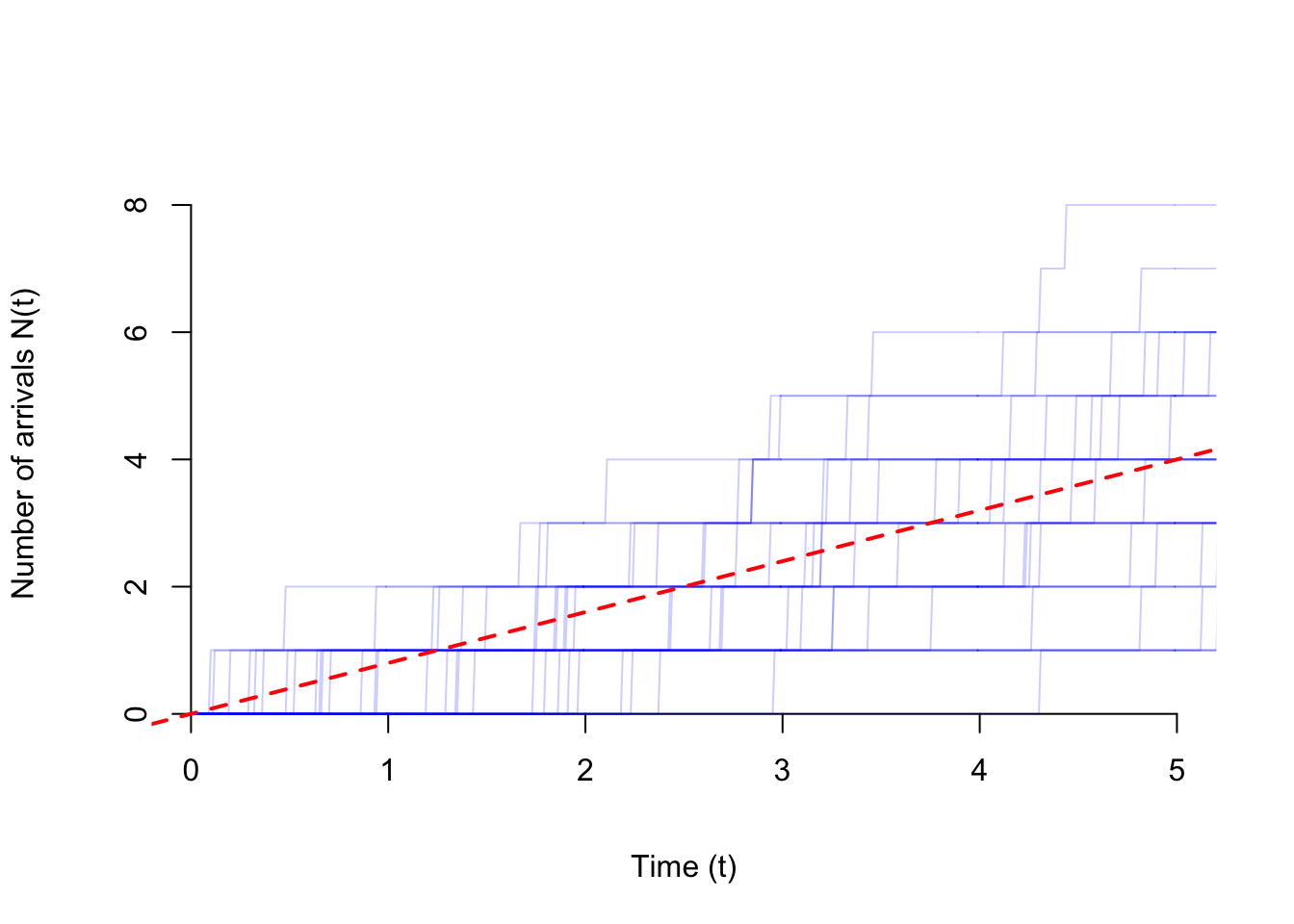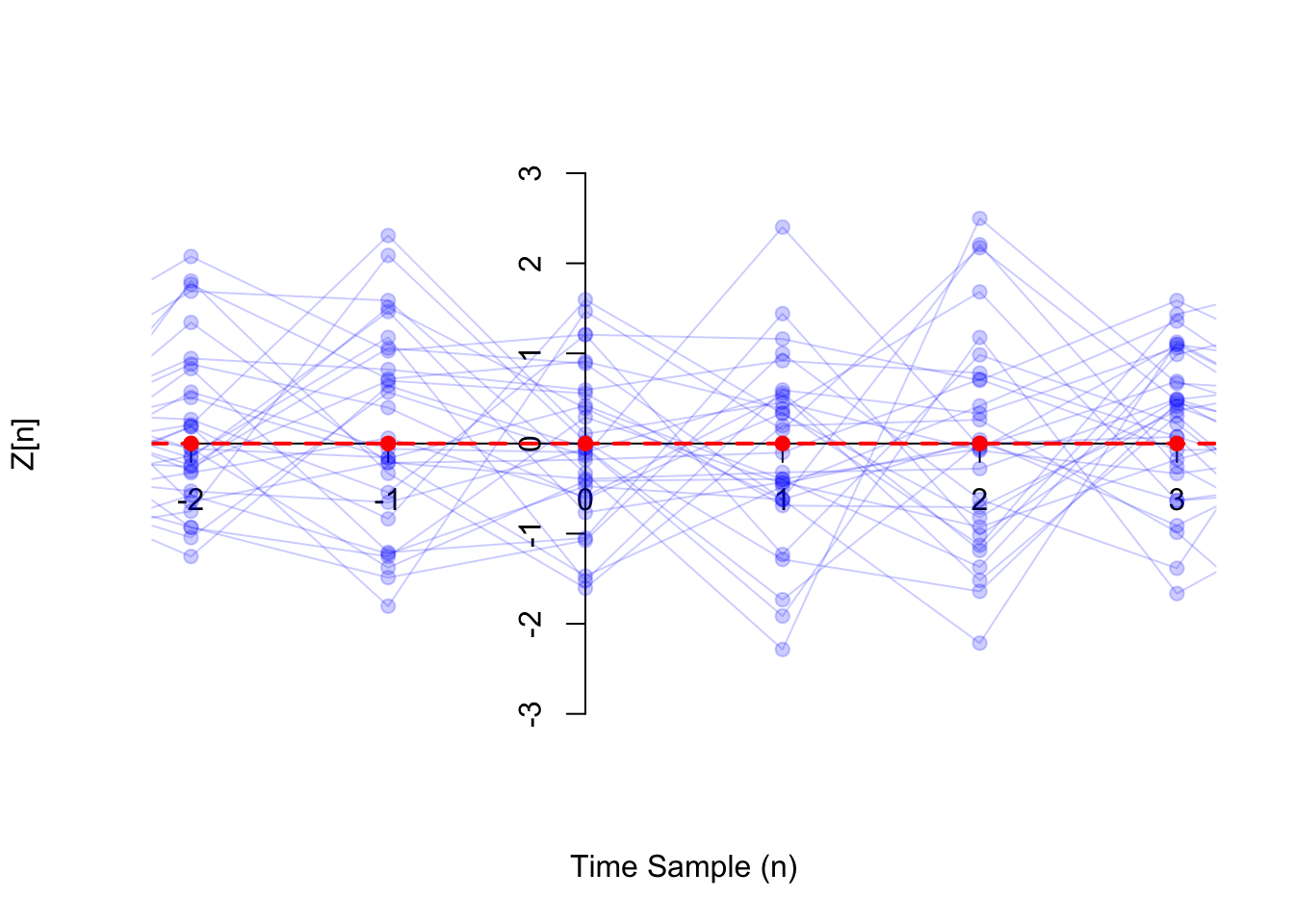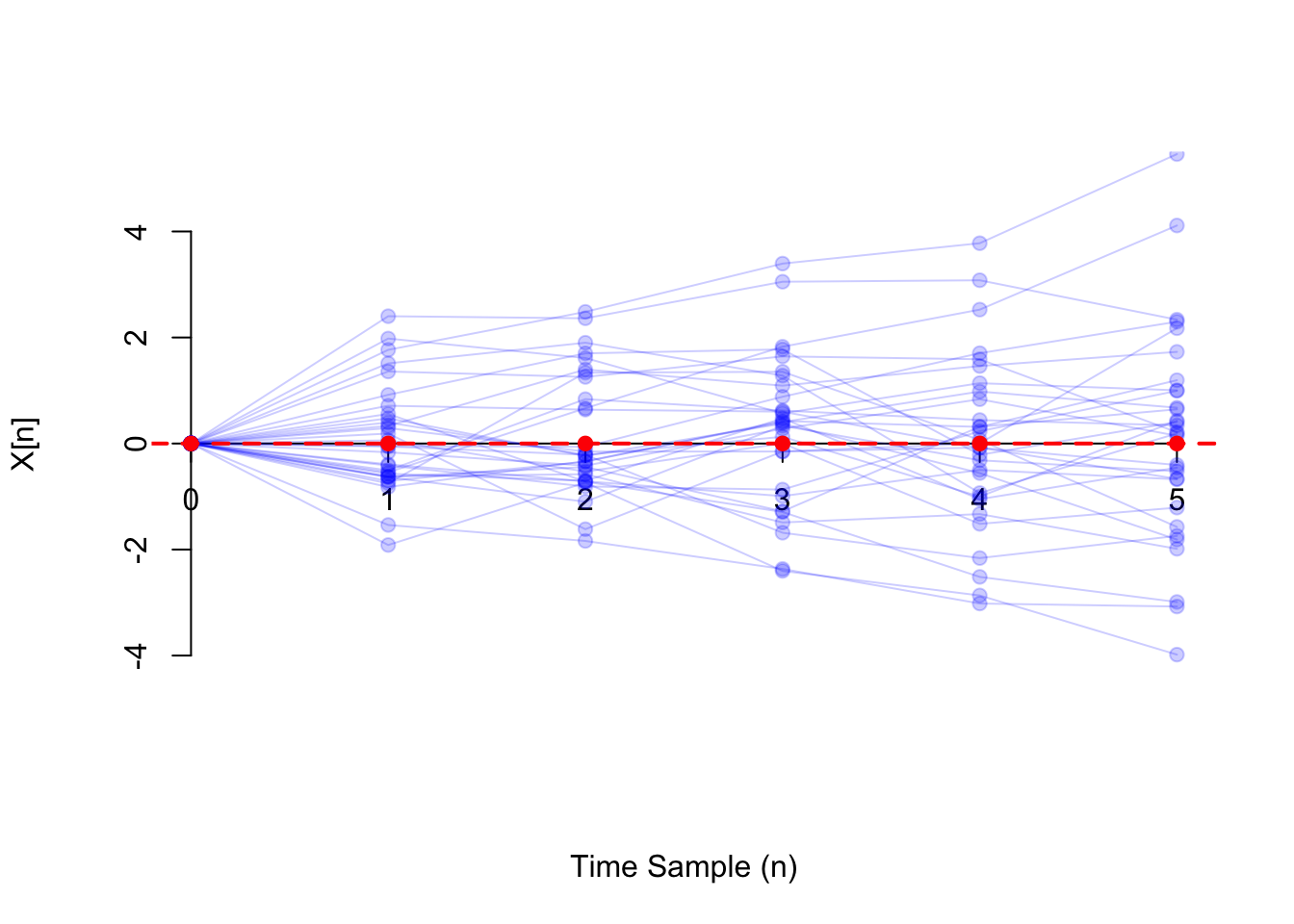# Lesson 50 Mean Function

In the next few lessons, we discuss three functions that are commonly used to summarize random processes: the mean function, the variance function, and the autocovariance function.

## Theory

Definition 50.1 (Mean Function) The mean function $$\mu_X(t)$$ of a random process $$\{ X(t) \}$$ is a function that specifies the expected value at each time $$t$$. That is, $\begin{equation} \mu_X(t) \overset{\text{def}}{=} E[X(t)]. \tag{50.1} \end{equation}$

For a discrete-time process, we notate the mean function as $\mu_X[n] \overset{\text{def}}{=} E[X[n]].$

The following video explains how to think about a mean function intuitively.

Let’s calculate the mean function of some random processes.

Example 50.1 (Random Amplitude Process) Consider the random amplitude process $\begin{equation} X(t) = A\cos(2\pi f t) \tag{50.2} \end{equation}$ introduced in Example 48.1.

To be concrete, suppose $$A$$ is a $$\text{Binomial}(n=5, p=0.5)$$ random variable and $$f = 1$$. To calculate the mean function, observe that the only thing that is random in (50.2) is $$A$$. Everything else is a constant, so they can be pulled outside the expectation. \begin{align*} \mu_X(t) = E[X(t)] &= E[A\cos(2\pi ft)] \\ &= E[A] \cos(2\pi ft) \\ &= 2.5 \cos(2\pi ft), \end{align*} where in the last step, we used the formula for the expected value of a binomial random variable, $$E[A] = np = 5 \cdot 0.5 = 2.5$$.

Shown below (in blue) are 30 realizations of this process, along with the mean function (in red). Notice how the mean function passes through the heart of the realizations.Example 50.2 (Poisson Process) Consider the Poisson process $$\{ N(t); t \geq 0 \}$$ of rate $$\lambda$$, defined in Example 47.1.

Recall that $$N(t)$$ represents the number of arrivals on the interval $$(0, t)$$, which we know (by properties of the Poisson process) follows a $$\text{Poisson}(\mu=\lambda t)$$ distribution. Since the expected value of a $$\text{Poisson}(\mu)$$ random variable is $$\mu$$, we must have $\mu_N(t) = E[N(t)] = \lambda t$

Shown below (in blue) are 30 realizations of the Poisson process, with
the mean function superimposed (in red).Example 50.3 (White Noise) Consider the white noise process $$\{ Z[n] \}$$ defined in 47.2, which consists of i.i.d. random variables. Suppose the expected value of these random variables is $$\mu \overset{\text{def}}{=} E[Z[n]]$$. Then, the mean function is constant, equal to this expected value. $\mu_Z[n] = E[Z[n]] \equiv \mu.$

Shown below (in blue) are 30 realizations of a white noise process consisting of i.i.d. standard normal random variables, along with the mean function (in red), which in this case is $$\mu_Z[n] \equiv 0$$.Example 50.4 (Random Walk) Consider the random walk process $$\{ X[n]; n\geq 0 \}$$ of Example 47.3. Since $X[n] = Z + Z + \ldots + Z[n],$ the mean function is \begin{align*} \mu_X[n] = E[X[n]] &= E[Z] + E[Z] + \ldots + E[Z[n]] \\ &= n E[Z]. \end{align*}

Shown below (in blue) are 30 realizations of the random walk process where the steps $$Z[n]$$ are i.i.d. standard normal, so the mean function (shown in red) is $$\mu_X[n] = n \cdot 0 \equiv 0$$.## Essential Practice

1. Consider a grain of pollen suspended in water, whose horizontal position can be modeled by Brownian motion $$\{B(t)\}$$ with parameter $$\alpha=4 \text{mm}^2/\text{s}$$, as in Example 49.1. Calculate the mean function of $$\{ B(t) \}$$.

2. Radioactive particles hit a Geiger counter according to a Poisson process at a rate of $$\lambda=0.8$$ particles per second. Let $$\{ N(t); t \geq 0 \}$$ represent this Poisson process.

Define the new process $$\{ D(t); t \geq 3 \}$$ by $D(t) = N(t) - N(t - 3).$ This process represents the number of particles that hit the Geiger counter in the last 3 seconds. Calculate the mean function of $$\{ D(t); t \geq 3 \}$$.

3. Consider the moving average process $$\{ X[n] \}$$ of Example 48.2, defined by $X[n] = 0.5 Z[n] + 0.5 Z[n-1],$ where $$\{ Z[n] \}$$ is a sequence of i.i.d. standard normal random variables. Calculate the mean function of $$\{ X[n] \}$$.

4. Let $$\Theta$$ be a $$\text{Uniform}(a=-\pi, b=\pi)$$ random variable, and let $$f$$ be a constant. Define the random phase process $$\{ X(t) \}$$ by $X(t) = \cos(2\pi f t + \Theta).$ Calculate the mean function of $$\{ X(t) \}$$. (Hint: LOTUS)